# Catch up on the latest AI articles# Proposed A New Task Called Deep Imbalanced Regression (DIR), Which Is A Real-world Problem Faced By The Author

3 main points
✔️ Proposed a new task called Deep Imbalanced Regression(DIR)
✔️ Proposed a new method called LDS and FDS
✔️ Constructed 5 new DIR datasets

written by Yuzhe YangKaiwen ZhaYing-Cong ChenHao WangDina Katabi
(Submitted on 18 Feb 2021 (v1), last revised 13 May 2021 (this version, v2))

Subjects: Machine Learning (cs.LG); Artificial Intelligence (cs.AI); Computer Vision and Pattern Recognition (cs.CV)code：The images used in this article are either from the paper or created based on it.

## first of all

Looking at real-world data, it is often the case that the number of observations of a particular target is remarkably small and shows an unbalanced distribution. Learning on such unbalanced data has been studied extensively. And most of the studies in such cases often assume categories with clear boundaries. That is, most of the existing methods for dealing with unbalanced data/long-tail distributions often only address classification problems where the target is a discrete value in a different class. However, there are many real-world tasks where the targets are continuous.

The authors propose learning methods in data/long-tail distributions where such targets are continuous values and unbalanced and construct five new benchmark DIR datasets covering unbalanced regression tasks in computer vision, natural language processing, and medical problems. Then, we introduce our main contributions.

• We propose a new task called Deep Imbalanced Regression (abbreviated DIR), which we define as learning from imbalanced data with continuous targets that can be generalized to the entire target range.
• To solve the problem of learning from imbalanced data with continuous targets, we propose two new imbalance regression methods: label distribution smoothing (LDS) and feature distribution smoothing (FDS). proposed
• Building five new DIR datasets covering computer vision, natural language processing, and medicine to facilitate future research on imbalanced data

Data imbalance is ubiquitous in the real world, with skewed distributions with long tails rather than ideal uniform distributions in each category, and with significantly fewer observations at certain target values. This poses a significant challenge to the model, and some argue that this unevenness problem hinders the use of machine learning in the real world.

The learning techniques that have been used can be broadly classified as database and model-based methods. Data-based solutions include oversampling minority categories and undersampling the majority. Model-based methods include the use of specific relevant learning techniques, such as loss function reweighting, transfer learning, and meta-learning. However, existing methods for learning from unbalanced data mainly deal with discrete target values, as already mentioned above.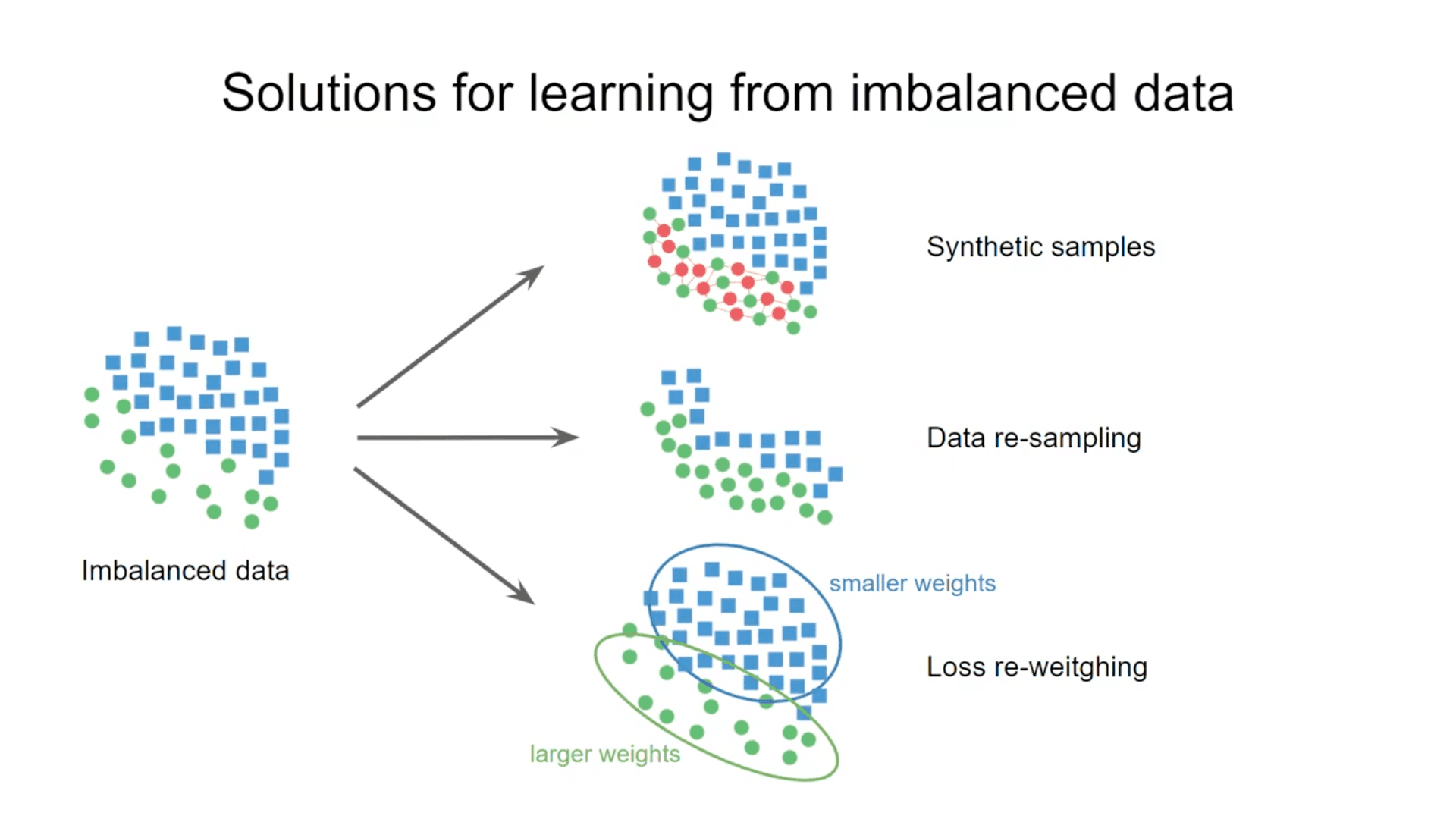For example, how about age estimation from faces? Age is a continuous value, and we expect it to be very biased within the target range. That's why you can expect less training data for babies and older people.

## Deep Imbalanced Regression: DIR

Deep Imbalanced Regression (DIR) aims to learn from imbalanced data with continuous target values, to address potential missing data in specific regions, and to allow the final model to generalize to the full range of target values.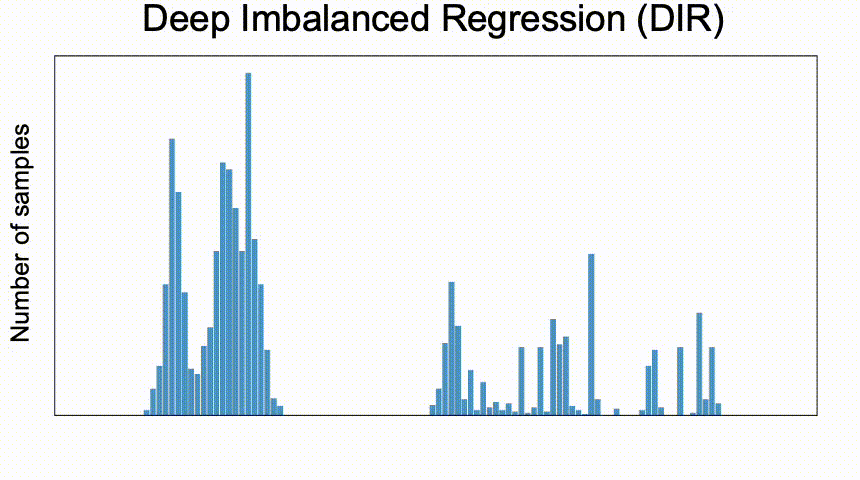## Difficulties and challenges

It is important to note that, compared to the unbalanced classification problem, DIR poses a whole new set of challenges, including

1. Given a continuous target value, there is no hard boundary between classes. Therefore, conventional methods such as resampling and reweighting cannot be applied directly.
2. Continuous labels make sense for the distance between targets. These targets tell us directly which data are closer together and which are further apart. This meaningful distance also provides further guidance on how we should understand the degree of imbalance in the data for this continuous interval.
3. Unlike the classification problem, in DIR there may be no data at all for the target value. Therefore, it is necessary to extrapolate or interpolate the target value.

Summarizing the above issues, it is clear that DIR poses new difficulties and challenges compared to the traditional problem setting. So, how should we perform DIR? In the next two sections, we will discuss Label distribution smoothing (LDS) and Feature distribution smoothing (FDS) to improve the model by exploiting the similarity between neighboring targets in label and feature space, respectively. smoothing (FDS) to improve models using similarity between neighboring targets in label and feature space, respectively.

## proposed method

### Label distribution smoothing (LDS)

To illustrate the difference between classification and regression problems in the presence of data imbalance, we present a simple example, using two different datasets, CIFAR-10 (a 100-class classification dataset) and IMDB-WIKI (a large image dataset for estimating age from human appearance). (a large image dataset for age estimation from human appearance). These two datasets are essentially completely different label spaces: CIFAR-100 is a categorical label space, i.e. its value is the class index; IMDB-WIKI is a continuous label space, i.e. its value is the age. To ensure that both datasets have the same range of labels, we restricted the age range of IMDB-WIKI to 0-99. Furthermore, to simulate imbalance in the data, we sampled both datasets so that the density distribution of the labels is exactly the same, as shown in the figure below (blue bars). They are essentially completely different datasets, but we just matched the density distributions of the labels.

We then train the ResNet-50 model on the two datasets and plot the test error distribution (red bars). From the left side of the figure, we can see that the error distribution is correlated with the label density distribution. Specifically, the test error as a function of the class index shows a high negative Pearson correlation (-0.76) with the label density distribution in the category label space. This phenomenon is easily predicted by the fact that classes with a large number of samples are learned better than classes with a small number of samples.

Interestingly, however, as shown on the right side of the figure, IMDB-WIKI shows a very different error distribution in continuous label space, even though the label density distribution is the same as in CIFAR-100. In particular, the error distribution is much smoother and less correlated with the label density distribution (-0.47). This example seems interesting because directly or indirectly, all imbalance learning methods work by compensating for imbalances in the empirical label density distribution. This works well for class imbalances, but for continuous labels, it means that the empirical density cannot accurately reflect the imbalance seen by the neural network. Therefore, correcting for imbalances in the data based on empirical label densities is inaccurate in continuous label space.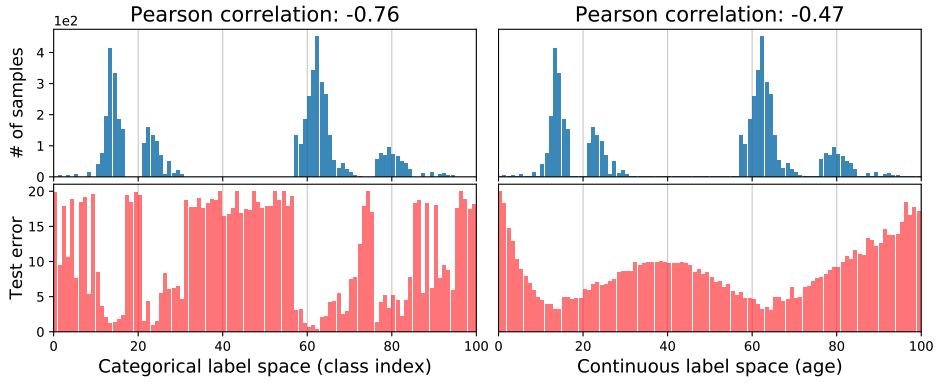Based on the results, we propose label distribution smoothing (LDS) to estimate the label density distribution, which is valid for the case of continuous labels. The approach in this paper uses the idea of kernel density estimation in the field of statistical learning to estimate the expected density. Specifically, given a continuous empirical label density distribution, LDS uses asymmetric kernel distribution function k with a convolution of the empirical density distribution to obtain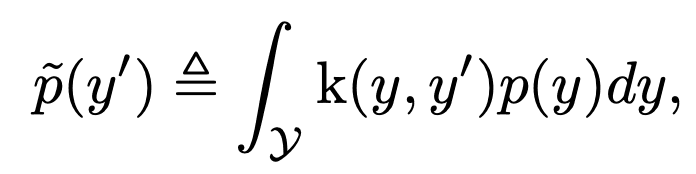We can confirm that the Pearson correlation coefficient between the effective label density distribution and the error distribution calculated by LDS is -0.83, which is well correlated. This means that by using LDS, we can create an imbalanced label distribution that actually affects the regression problem.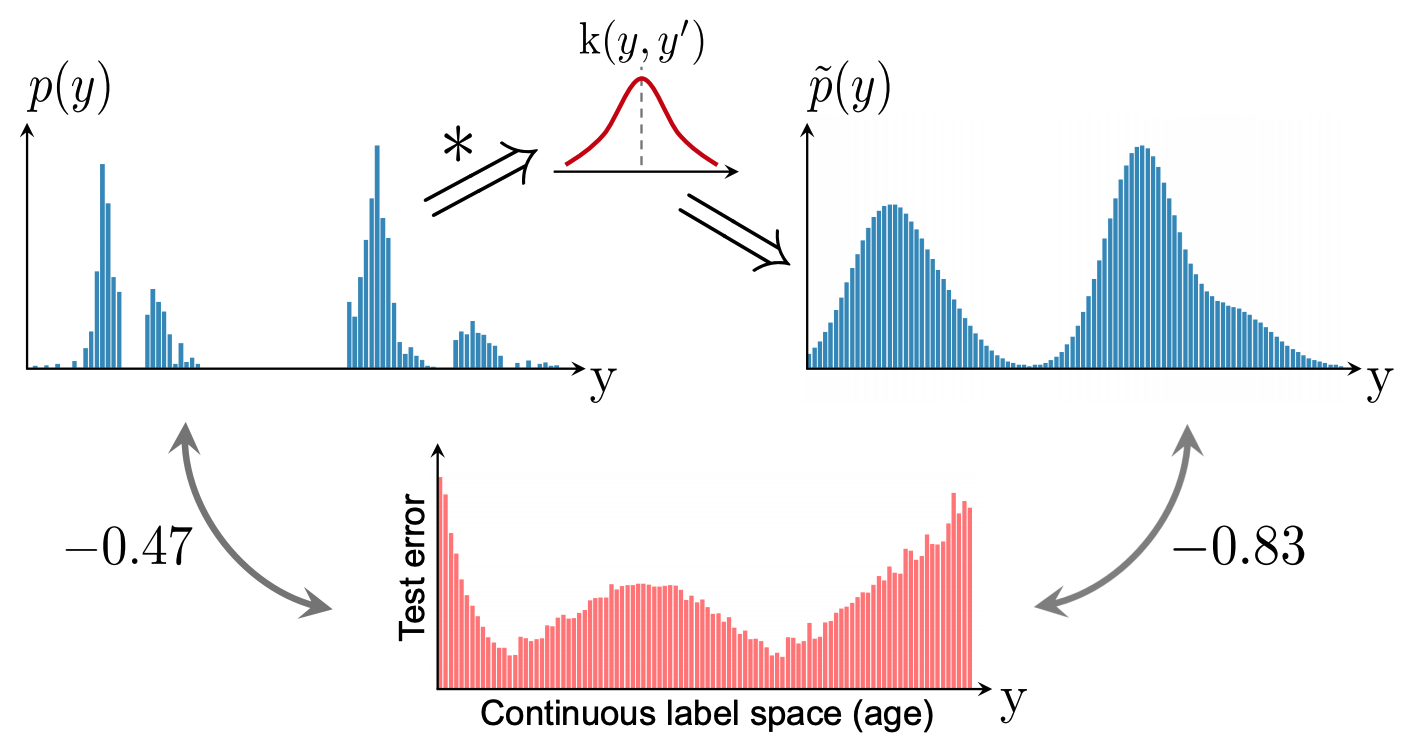### Feature distribution smoothing (FDS)

An overview diagram of the FDS is shown below.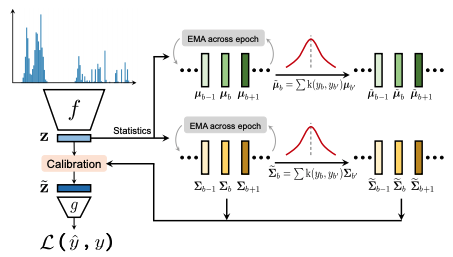This FDS is motivated by the intuition that continuity in the target space should produce continuity in the corresponding feature space. In other words, if the model is working correctly and the data are balanced, then the statistics of features corresponding to nearby targets should be close to each other. To show how data imbalance affects DIR features, we will use a model trained on images from the IMDB-WIKI dataset to infer the age of a person based on their appearance. We compute the statistics (mean and variance) of the features with respect to each data, which we denote as {$µ_b,σ_b$}. To visualize the similarity of the features, we select a particular data and calculate the cosine similarity of the features with all other data.

The results are shown in the figure below; for 30, the regions with different data densities are shown in purple, yellow, and pink colors. From the figure, we can see that the statistics of the features around 30 are very similar. Specifically, the cosine similarity of all feature means and feature variances from ages 25 to 35 are within a few percent of their values at age 30. Thus, the figure confirms the intuition that features statistics are similar when there is sufficient data or for consecutive targets.

Interestingly, the figure also shows problems in areas where the sample size of the data is very small, such as the age range from 0 to 6 years (pink). Notice that the mean and variance values for this range show an unexpectedly high similarity to the 30-year-olds. In fact, it is shocking that the feature statistic for age 30 is more similar to age 1 than to age 17. This unwarranted similarity is due to imbalances in the data.

Anyway, using age as an example, close ages should be similar and far ages should not be similar! But the reality is that the data is biased and strange things are happening.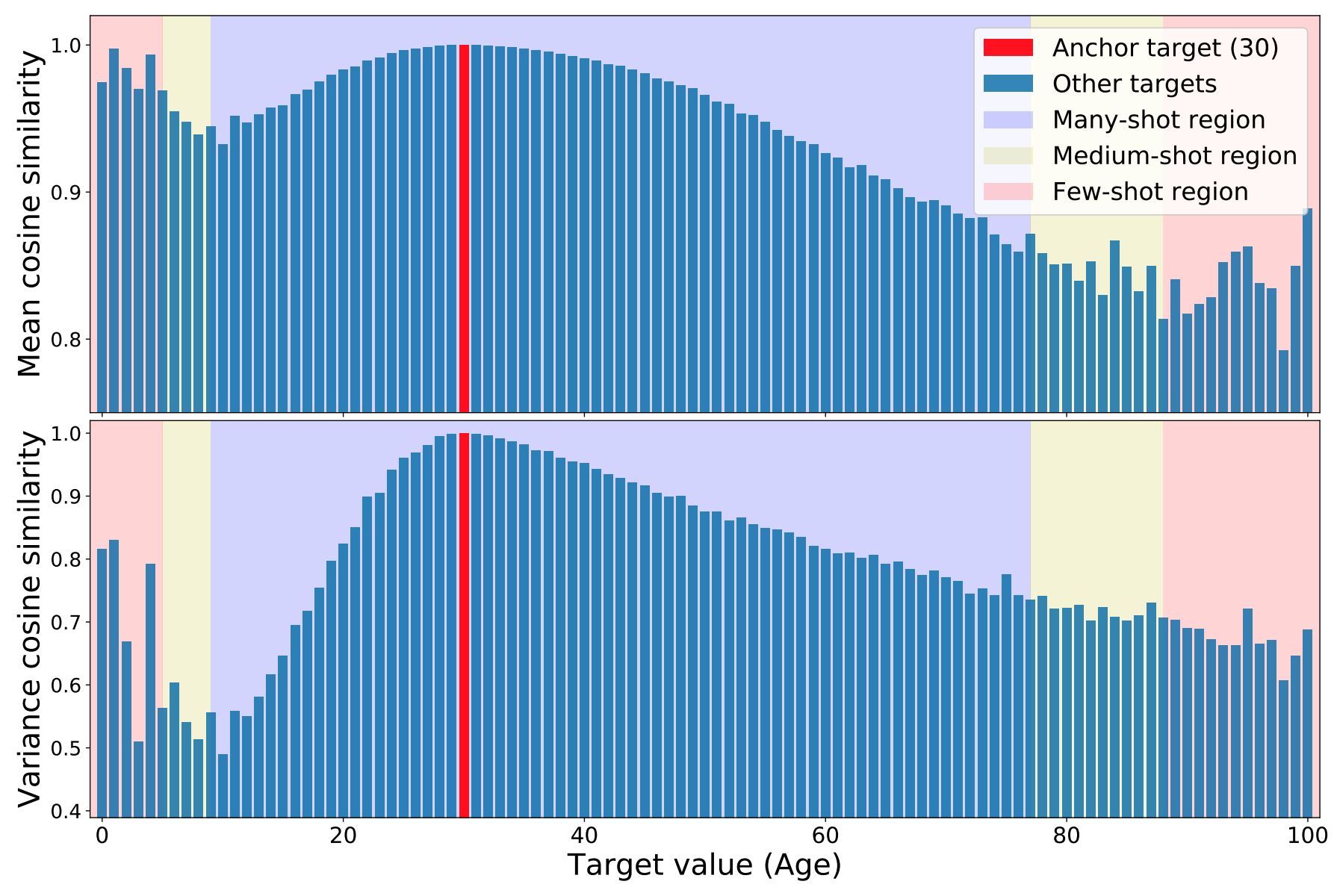It performs distribution smoothing in the FDS feature space, moving feature statistics between nearby targets. FDS is performed by first estimating each statistic and then replacing the variance with a covariance to reflect the relationship between the various feature elements in z, without loss of generality. The variance is replaced by the covariance in order to.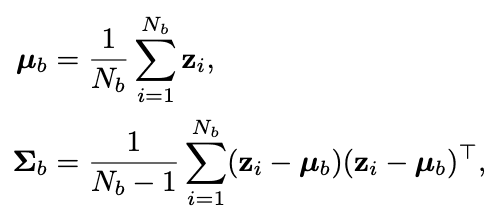$N_b$ is the bth sample number. Given the statistics of a feature, we again employ the symmetric kernel k($y_b$,$y_b'$) to smooth the distribution of the mean and covariance of the feature on the data of interest. This yields a smoothed version of the statistic.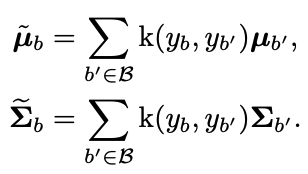The general statistics and the smoothed statistics, we then follow standard whitening and recoloring procedures to calibrate the feature representation of each input sample.

We integrate the FDS into the model by inserting a feature calibration layer after the final feature map. To train the model, we employ a momentum update of the running statistics over each epoch, and correspondingly the smoothed statistics are updated across different epochs but fixed within each training epoch.FDS can be used with any model as well as previous work to improve label imbalance It can be integrated. In fact, in the paper, we demonstrate that integrating our method with methods to improve other imbalance problems can consistently improve performance.

## The DIR data set, a benchmark

In dataset DIR, we have five benchmarks: computer vision, natural language processing, and healthcare. The figure shows the label density distributions of these datasets and their degree of imbalance. (For more details on the data, please refer to the original publication)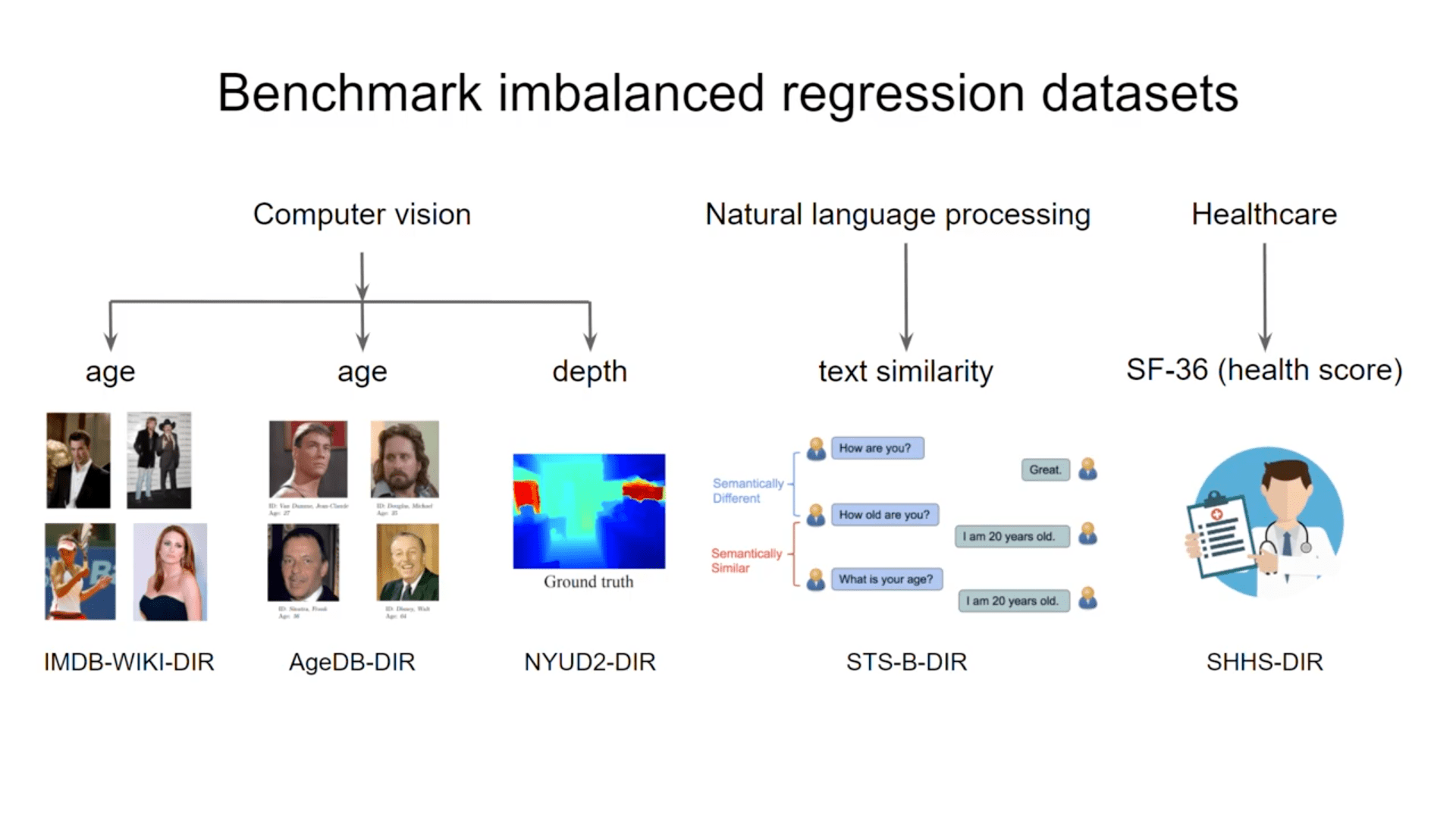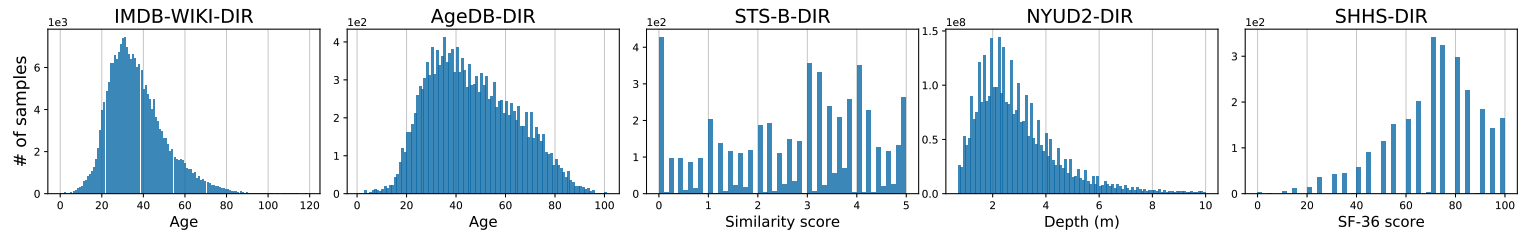• IMDB-WIKI-DIR
IMDB-WIKI-DIR is constructed from the IMDB-WIKI dataset, which contains 523.0K face images and their corresponding ages.
• AgeDB-DIR
AgeDB-DIR is built from the AgeDB dataset.
• STS-B-DIR
We build STS-B-DIR from the Semantic Textual Similarity Benchmark, which is a collection of news headlines, video and image captions, and sentence pairs extracted from natural language inference data.
• NYUD2-DIR
NYUD2-DIR is built from NYU Depth Dataset V2.
• SHHS-DIR
The SHHS dataset contains all-night polysomnography (PSG) for 2651 subjects; PSG signals include EEG, ECG, and respiratory signals (airflow, abdominal, and chest). The dataset includes a 36-item Short-Form Health Survey (SF-36) for each subject, from which general health scores are extracted.

## experimental results

Here we only show the results of IMDB-WIKI-DIR, which is mentioned as the main result. For details of all results, please refer to the original publication. As shown below, we compare the results with different methods and apply each LDS, FDS, and the combination of LDS and FDS to the baseline method. Finally, we report the performance improvement of LDS + FDS over the vanilla model. As shown in the table, LDS and FDS perform very well regardless of the type of learning method used, with improvements of several tens of percent.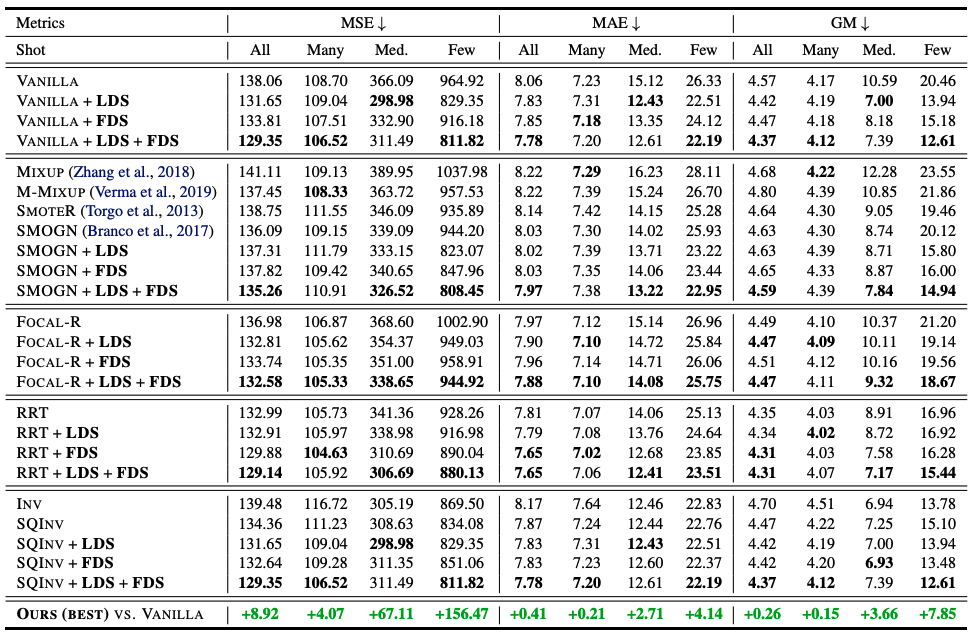### consideration

There is a more detailed analysis of why FDS works. The first is an analysis of how FDS affects the learning process of the network. We show a similarity plot of the feature statistics for base age 0. As can be seen, the small sample size of the target interval, age 0, causes a large bias in the feature statistics. For example, the statistical values for age 0 are also supposed to be similar for the interval from 40 to 80 years old.

On the other hand, when FDS is added, the statistics are better calibrated and only their surroundings have a high similarity, with the similarity decreasing as the target value increases. Furthermore, it is interesting to visualize the $L_1$ distance between the running and smoothed statistics during training: the average $L_1$ distance decreases as training progresses and converges to 0. This indicates that without smoothing, the model learns to produce more accurate features and eventually produces good results in the inference process, i.e. without using the smoothing module.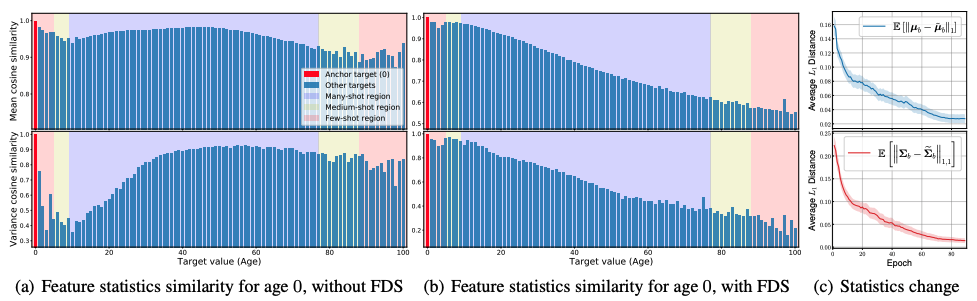## summary

DIR is systematically studied and correspondingly simple and effective new methods LDS and FDS are proposed to solve the problem of learning imbalanced data with continuous goals and establish five new benchmarks to facilitate future regression studies on imbalanced data. The paper provides a very intuitive problem analysis and explanation.

In fact, the author of this paper is running a separate project related to healthcare AI. The author defines the current problem from the experience that label distribution is very sparse and biased with continuous values, and finally proposes LDS and FDS. It is found that the performance can be significantly improved compared to the baseline model, which validates the effectiveness and practicality of the method in real-world tasks, and it is hoped that the method will not be limited to academic data sets.

However, due to the use of symmetric kernels as a future challenge, there is a problem of hyperparameterization and the optimal parameters may vary from task to task. We need to determine the appropriate values based on the labeling space of a particular task. However, I think this paper is a great starting point to define the problem for this issue.

If you have any suggestions for improvement of the content of the article,